Technische Universität Wien
Institut für Festkörperphysik

## Nano-Systems

Quantum dots are nano devices, where quantum effects play a decisive role. Our research focuses on studying such quantum effects in single or coupled quantum dots allowing a realistic calculation of statistical properties of quantum dots. Particularly, we are interested in the Kondo effect (which is also important for dynamical mean-field theory), correlations between dots, and the search for dephasing mechanisms and effects.

### Mesoscopic/Nano Physics

Conventionally, solid state physics deals with macroscopically large systemswhere the system sizeis much larger than the typical scattering lengthduring which momentum (or phase information) of the electrons is lost, and both are much larger than the atomic length scale given by the Bohr radius. In this case, the macroscopic sample can be divided into many subsystems and physical properties (e.g., the conductivity) are calculated by a statistical average over the subsystems, such that they are independent of the particular scatterer distribution, see Fig. 1.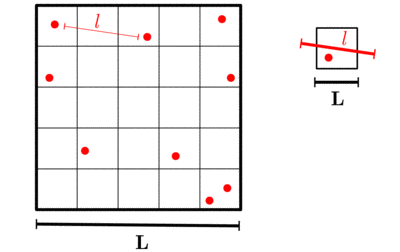Figure 1: Macroscopic and... ...mesoscopic world.

With the further miniaturization in semiconductor physics, the parameter regime, where the sample size in one or more dimension becomes similar to (or even smaller than) the scattering length is reached:In this case we talk about a mesoscopic system. In a mesoscopic system, there is no statistical average over many subsystems anymore and the physical properties (e.g. the conductance through the system) become sample dependent, though sample-to-sample fluctuations can reveal universal statistical behavior. In such mesoscopic or nano systems with a confined geometry, quantum mechanical effects become much more important. Today with(or), we are in between the mere macroscopic and the mesoscopic (or nano) world. Engineers are still able to build computer chips on the basis of classical, macroscopic calculations (only some input parameters depend on quantum mechanics, like the semiconducting gap which depends on the exchange interaction). On the other hand, physicists are already able to explore the quantum effects of the new mesoscopic or nano world.

In the area of mesoscopic or nano physics, our research focuses on single and coupled quantum dots, wherein all three dimensions, see Fig. 2. The Coulomb interaction between the electrons in the quantum dot can be employed as an energy barrier, so that exactly an integer number of electronsis located on the dot. Because it is not possible to add or remove electrons, the dot is Coulomb blockaded and the current through it is zero, or negligibly small. If we increase the gate voltage, we will attract more electrons, and the dot is Coulomb blockaded withelectrons, except for a very particular gate voltage, at which the energy for the configurations withandelectrons is degenerate. For this particular voltage and in a small window around it, electrons can be moved onto the dot and there is a current through the dot. In this way, the quantum dot can act as a single electron transition, where we control the current by the gate voltage.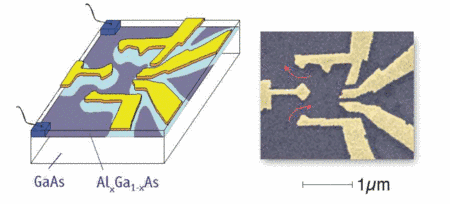Figure 2: A semiconductor quantum dot as investigated by our experimental colleagues. The current flows from the source to the drain electrode with the gate electrodes controlling the tunneling amplitudes and the number of electrons in the dot.

Theoretically, we describe the quantum dot (see Fig. 3) by the Hamiltonian,

where the (isolated) dot and the Coulomb repulsion between the electrons in the dot are described by,

and,

while the environment (leads) and its hybridization with the dot is accounted for in the terms.

and,

The Hamiltonian above contains the energy levelsof independent electrons via the eigenvalues of amatrix, withbeing the creation (annihilation) operator for an electron in the dot (leads), the Coulomb interaction (charging energy)between all electronson the dot, the gate voltage. These charging () and single particle term () describe the isolated dot. It couples to the leads via a term () describing the hopping of an electron from the dot to the left () and right () electrode (term) and vice versa (). Finallydescribes electrons in the electrodes by the linearized eigen energiesand a possible voltage differencebetween left and right electrodes. With so many parameters, we either study the generic effects qualitatively or employ random matrix theory to describe the parameters statistically.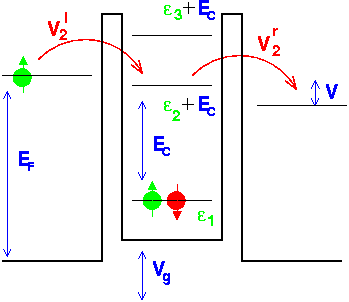Figure 3: Theoretical sketch of a quantum dot.

### Random matrix theory (RMT)

In statistical mechanics, we deal with large, macroscopic samples so that we cannot keep track of the microscopic state the system is in. With the lack of knowledge, the best thing we can do is to describe the system statistically, assuming that all microscopic states with the correct energy and symmetry are equally probable. And it works! In random matrix theory, we go a step beyond. We assume that the Hamiltonian (3) with the myriad of parameters is itself statistically distributed, with different dots corresponding to different statistical realizations. The matrixhas random entries just following the basic symmetries of our system. Without (or with) an external magnetic field, we have (or have not) time-reversal symmetry, and the elements are real (complex). If the matrix is big enough (), the statistical properties of, e.g., the distance between consecutive eigenvalues follows the universal statistical behavior of the Gaussian Orthogonal or Unitary Ensemble (andrespectively). Moreover, one can even show that this statistical RMT description becomes exact(!) for large quantum dots and is controlled in a parameter (, whereis the number of levels in the dot), which is small for quantum dots with a typical area of the order to a. From the RMT for the dot we can also calculate the hopping elements to the leads, which are just given by the overlap of the quantum dot’s eigenvectors with a vector describing the conducting channel to the leads. Furthermore, in leading order inthe charging energydoes not depend on which levels the electrons occupy.

### Dephasing

A genuine quantum effect is that an electron has, besides its momentum, also a phase. To study the quantum effects due to this phase and the mechanisms, by which the electron loses its phase, is of fundamental theoretical interest. To understand dephasing, let us consider the following (gedanken) experimental setup: we have prepared a bunch of electrons (or, for the sake of simplicity, just one electron) with a fixed phase. Now, this electron is injected into a bigger system, for which we have no control of the phase (there are just too many electrons). If the electron is only scattered elastically at some disorder potential (see right part of Fig. 4), the phase of final statehas a fixed phase relation with the initial state, given by the one-electron Schrödinger equation:. While the elastic scattering process leads to a loss of momentum, phase information is kept. On the other hand, if we consider inelastic scattering processes (e.g., electron-electron interaction, depicted on the right hand side of Fig. 4), the electron couples to an exchange boson, which also has a phase. This phase is given by the big system and uncontrolled (or random). Hence, the phase of the final state now depends on the phase of the initial state and the uncontrolled phase of the exchange boson. The phase information from the initial state is lost.

We are interested in the effects of such dephasing and its microscopic origin and description, which is still an exciting puzzle. So far, we have been able to calculate dephasing times from magneto-conductance experiments in nearly-isolated quantum dots, based on a phenomenological theory.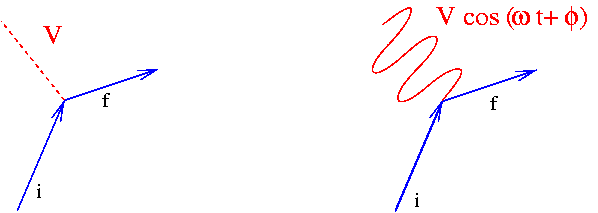Figure 4: Elastic... and inelastic scattering: Losing momentum... and momentum/phase information.

### Manganites nanoclusters

Manganese-based perovskite oxides are the prototypical materials exhibiting a colossal magneto resistance, i.e., the dramatic change in the resistance of a material upon the application of an external magnetic field. The origin of the phenomenon is still debated; as as the microscopic origin have been proposed the interplay between polarons and electronic correlations for low doping levels [Y.-F. Yang and K. Held, Phys. rev. B 76, 212401 (2007); Phys. Rev. B 82, 195109 (2010)]. The enormous complexity of manganite compounds displays a rich phase diagram as a function, e.g., of temperature and doping concentration (below indicated as). In particular we investigated the half-doped LaCaMnOwhich is at the edge of a strongly first order transition between two phase characterized by completely different order (i.e., charge-orbitally ordered insulating and ferromagnetic metallic). Hence, it is particularly susceptible to external perturbations. It was experimentally shown that the insulating charge-orbital order is destabilized, in favor of a FM metallic phase, by various means, including doping, pressure, electric and magnetic field. Recently, a few experimental studies reported the destabilization of the CO order even due to size reduction. Size control of, e.g., the magnetoresistive properties of materials, is appealing from the technological point of view, because nano-crystal can be engineered at low-cost by means of chemical methods.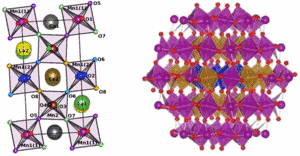Left: unit cell of bulk LaCaMnO. Right: nanoscopic cluster of aboutnm size.

In order to get a theoretical understanding of the size-driven transition, we investigated both bulk and nanoscopic LaCaMnO. Employing a combination of ab-initio density functional theory and dynamical mean-field theory, we take into account both the evolution of the crystal structure upon size reduction, as well as strong electronic correlations in the narrow Mn-orbitals. This way, we could show that (i) in the bulk, electronic correlations stabilize the ordered phase, and (ii) the change of the lattice parameters upon size reduction is the driving force undermining the complex charge-orbital order in LaCaMnO.

 H. Das, G. Sangiovanni, A. Valli, K. Held, and T. Saha-Dasgupta, Size Control of Charge-Orbital Order in Half-Doped Manganite LaCaMnO, Phys. Rev. Lett. 107 197202 (2011)